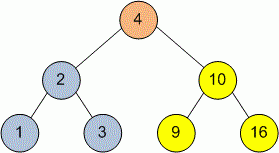Competitions

# Tree Maximum element

Binary search tree is given. Return the pointer to the maximum element.

Definition of a tree:

// Java
class TreeNode
{
public:
int val;
TreeNode left;
TreeNode right;
TreeNode(int x) {
val = x;
left = NULL;
right = NULL;
};

// C++
class TreeNode
{
public:
int val;
TreeNode *left;
TreeNode *right;
TreeNode(int x) : val(x), left(NULL), right(NULL) {}
};


Implement function Maximum that returns pointer to the element with maximum value in the tree.

// Java
TreeNode Maximum(TreeNode tree)

// C++
TreeNode* Maximum(TreeNode *tree)


#### ExampleFunction Maximum returns pointer to the node with value 16 - the vertex with maximum value in the tree.

Time limit 1 second
Memory limit 128 MiB
Author Mykhailo Medvediev